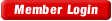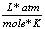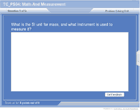HOME COURSES PREVIEW REVIEW ABOUT
 TOLL-FREE INFO & ORDERING: M-F: 9am-5pm (PST): (877) RAPID-10
 QUICK TOUR: Quick Movie - How to Learn in 24 Hours
 QUICK SEARCH: Keywords:
 Rapid Learning Member Area:Note: If you are a legacy user of chemistry24 members, please request a new login access to the premium server with your full name and old login email via vip@rapidlearningcenter.com
 RAPID COURSE CATALOG: Chemistry in 24 Hours
CHEMISTRY TIPS WEEKLY:

Are you taking a chemistry course or preparing for a chemistry exam? If you are learning chemistry now, the Chemistry Tips Weekly is for you--it is a one minute learning each week to the chemistry mastery, free for all students. The chemistry tips will include the follwing topics

• Chemistry survival basics
• Top ten confusions in chemistry
• How to ace chemistry exams
• Problem-solving tips in chemisrty
• Plus much more...
Enter your name and email address below and click "Subscribe" to have free Chemistry tips delivered to your inbox weekly.

 OTHER RELATED SITES: ChemistrySurival.com BiologySurvival.com Biology24.com Physics24.com Mathematics24.com RapidLearningCenter.com
 Note: For course links to launch, disable popup blockers or hold the ctrl key while clicking the link.Bonus - Free Coaching for 3 Months :  Need help on your homework or exam questions? Get stuck on chemistry problems? Let Dr. Wayne and his team of faculty help you personally. Order one of our 24-hour rapid chemistry courses today and you will receive 3-month chemistry coaching (\$297 value - free). Limited to first 250 students only. Grab yours today!

Measurement & Math in Chemistry

Topic Review on "Title":

Chemistry is an experimental science, therefore it is necessary to be able to work with units and measurements accurately.

Metric System
The metric system is based on prefixes that indicate a power of 10 with base units.

 Metric Prefixes commonly used in chemistry Prefix Symbol Multiple Kilo k 1000 Deci d 0.1 Centi c 0.01 Milli m 0.001 Micro m 0.000001 Nano n 0.000000001

SI System
The International System of units gives a standard unit for each type of measurement.

 SI Units commonly used in chemistry Measurement Unit Symbol Mass Kilogram kg Volume Liter L Temperature Kelvin K Length Meters m Time Seconds s Amount of substance Mole Mol Energy Joule J Charge Coulomb C

There are also some important non-SI units as well.

 Non-SI Units commonly used in chemistry Measurement Unit Symbol Length Anstrom Å Pressure Atmosphere Atm Kilopascal kPa Energy Calorie cal Temperature Celcius °C

Taking measurements
Measurements must be taken accurately.  Always write down one more decimal place than the instrument tells for certain—a “0” if it’s “one the line” and a “5” if it’s “between the lines.”

Significant Figures
The significant figure rules are to allow people to read data or calculations and know with what precision the data was taken.  The significant rules can be summarized in two rules: (1) If a decimal point is not present, count digits starting with the first the first non-zero number and ending with the last non-zero number; (2) If a decimal point is present anywhere in the number, start counting with the first non-zero number and continue until the end of the number.  Rules on how to perform calculations with significant figures will be given in a future tutorial.

Fundamental Constants
Several numbers are used throughout chemistry and are important to be familiar with.

 Fundamental constants commonly used in chemistry Name Symbol Constant Avogadro’s # NA 6.02 X 1023 mol-1 Speed of light c 3.0 X 108 m/s Gas constant R 8.310.0821Planck’s constant h 6.63 ´ 10-34 J·s Charge of electron e 1.6 ´ 10-19 C Atomic mass unit m 1.66 ´ 10-24 g Std Temp & Pressure STP 273.15 K & 1 atm

Math skills are needed throughout a chemistry course.

Algebra
Algebra is used to solve equations by un-doing whatever is being done to an unknown variable. For example, if an equation has “x+2” then you would subtract “2” to solve for “x”.  Everything that is done to one side must be done to the other side of the equation as well.

Calculations with significant figures
You cannot become more precise after completing calculations than the original data was.  Therefore, it is important to write the answer with the correct number of significant figures.  When adding and subtracting with significant figures, you write the answer with the least number of decimal places that are in the problem.  When multiplying and dividing, write the answer with the least number of significant figures as is in the problems.

Scientific Notation
Scientific notation is a way of writing large or small numbers as a multiple of 10.  The decimal place is always placed behind the first non-zero number and the number of times the decimal point was moved to get there is used as the exponent of 10.  Positive exponents represent large numbers (>1) and negative exponents represent small numbers (<1).

There are when working with scientific notation numbers:

• Addition with same powers of 10:  Add the numbers and keep the power of 10 the same.
• Subtraction with the same powers of 10:  Subtract the numbers and keep the power of 10 the same.
• Multiplication: Multiply the numbers and add the powers of 10
• Division: Divide the numbers and subtract the powers of 10
• Power: Take the number to that power and multiply the power of 10 by the power
• Roots:  Take the root of the number and divide the power of 10 by the root

Logarithms
Logarithms are a way of counting in multiples of a base number.  Ifthen.  If no base is specified, it’s assumed to be 10.

Calculator Tips

People often get incorrect answers simply from a mistake in the way they enter numbers into their calculator.  When dividing by more than one number, use the ¸ button each time.  When entering scientific notation, always use the EE (or EXP) button rather than entering (^10).  Be sure to use parenthesis around addition and subtraction when combining with multiplication and division, and also when taking a value (especially a negative value) to a power.

Rapid Study Kit for "Title":
 Flash Movie Flash Game Flash Card Core Concept Tutorial Problem Solving Drill Review Cheat Sheet"Title" Tutorial Summary : The Metric and SI (International System) of units is used throughout chemistry.  The metric system is based on prefixes showing the power of 10 used with base units describing the quantity measured. Chemistry is an experimental science; therefore it is necessary to take careful measurements.  Measurements should always include one more decimal place than the instrument indicates for certain—this last decimal place should be a “0” if the measurement is “on the line” and a “5” if the measurement is “in-between the lines.”  Most measurements are understood to be +/- 1 in the last decimal place, unless another uncertainty value is given with the measurement. Once careful measurements are taken, the precision with which they were measured can not be heightened while doing calculations, nor should it be lost and allowed to become less precise.  Therefore, there are rules about counting “significant figures” which indicate which were measured for certain.  Math skills are crucial throughout chemistry.  This tutorial reviews basic algebra needed in chemistry calculations.  Writing answers with the correct number of significant figures is taught, along with writing and reading numbers in scientific notation.  Performing calculations with exponents, including those in scientific notation, is illustrated.  Logarithms, both base 10 and natural logs, is taught.  The quadratic equation is demonstrated.  Finally, calculator survival tips are given to ensure the answer you type in what you intend.  All mathematical concepts are illustrated with chemistry applications—each one is actually used in chemistry calculations.

 Tutorial Features: Specific Tutorial Features: Examples of the math techniques use chemistry applications  Series Features: Concept map showing inter-connections of new concepts in this tutorial and those previously introduced. Definition slides introduce terms as they are needed. Visual representation of concepts Animated examples—worked out step by step A concise summary is given at the conclusion of the tutorial.

 "Title" Topic List: The Metric and SI Systems Metric prefixes SI units Common non-SI units Measurement and Uncertainty Tools common in Chemistry Labs Taking measurements Uncertainty Lab Safety Common Lab Procedures Significant Figures Why they’re used How to count them Fundamental Constants used in Chemistry Algebra Solving for a variable in addition/subtraction and multiplication/division Calculating with significant figures Addition/subtraction Multiplication/division Scientific Notation Writing in scientific notation Reading scientific notation Calculations with scientific notation Logarithms Logarithms with base 10 Calculator tips Tips for Studying Chemistry

See all 24 lessons in college chemistry, including concept tutorials, problem drills and cheat sheets:
Learn Yourself to Teach Chemistry Visually in 24 Hours

 © 2015 Rapid Learning Center | Privacy Policy | Disclaimer | Affiliates Chemistry Survival, Biology Survival, Physics Survival, and Mathematics Survival Publishing are the divisions of Rapid Learning Inc.